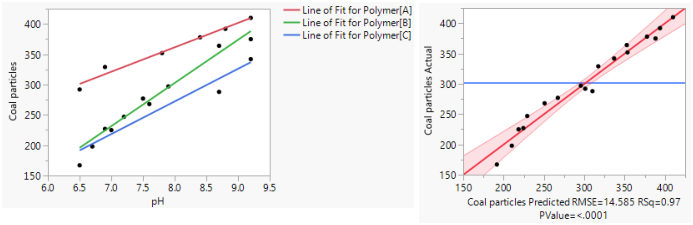Fitting Linear Models > Standard Least Squares Models
Publication date: 11/10/2021

# Standard Least Squares Models

##### Analyze Common Classes of Models

The Standard Least Squares personality within the Fit Model platform fits a wide spectrum of standard models. These models include regression, analysis of variance, analysis of covariance, and mixed models, as well as the models typically used to analyze designed experiments. Use the Standard Least Squares personality to construct linear models for continuous-response data using least squares or, in the case of random effects, restricted maximum likelihood (REML).

Analytic results are supported by compelling dynamic visualization tools such as profilers, contour plots, and surface plots (see Profilers). These visual displays stimulate, complement, and support your understanding of the model. They enable you to optimize several responses simultaneously and to explore the effect of noise.

Figure 3.1 Examples of Standard Least Squares Plots